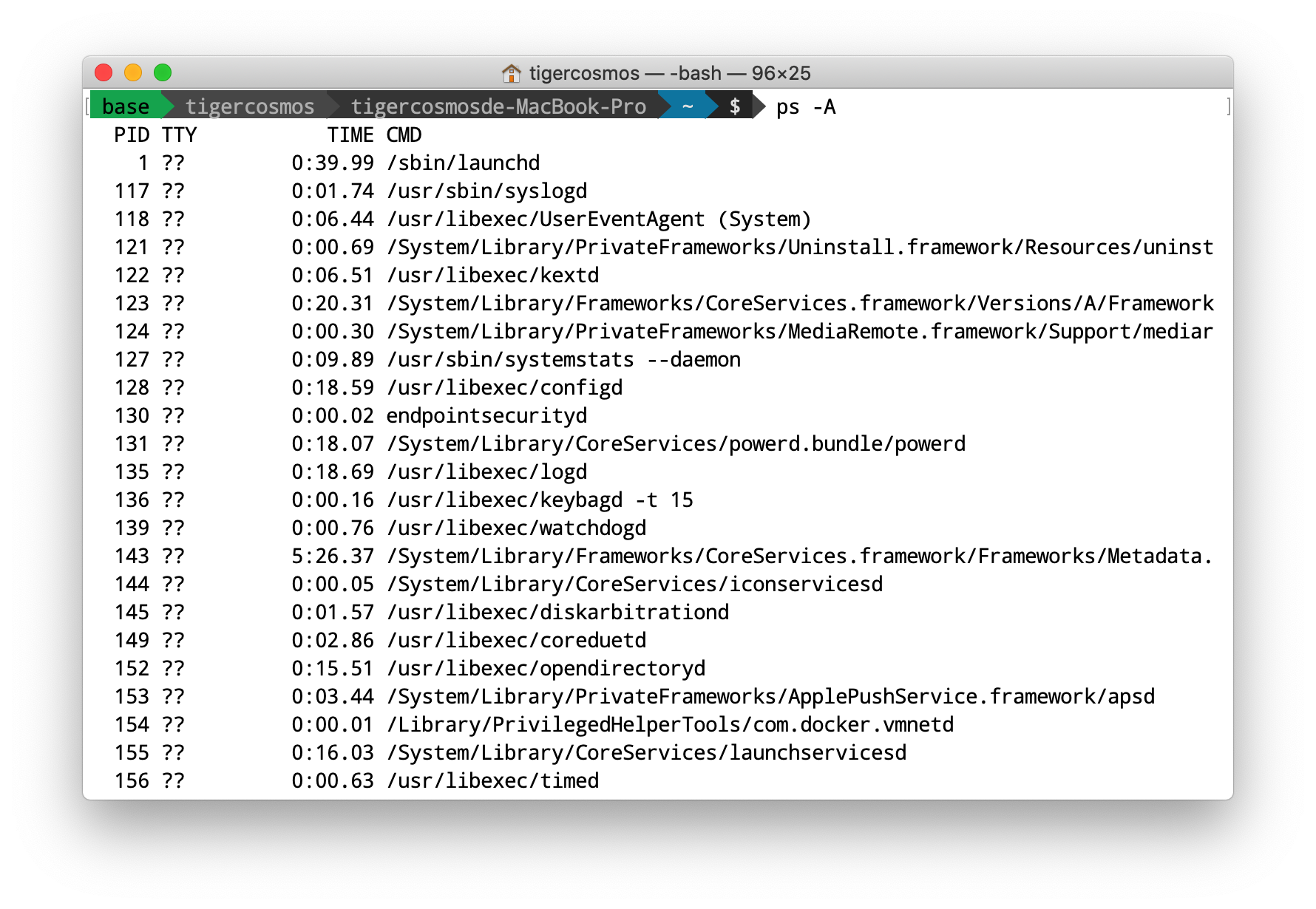## ¶ Shell 介紹Shell 最強的地方是，一些平常的工作可以快速解決，像是查詢目前 chrome 開了哪些執行序，就可以下 ps au | grep chrome，其中 ps 是列出程序，grep 是擷取符合 chrome 的文字。| 是「pipe」，A | B 代表將 A 輸出的東西丟給 B，B 會收取 input 然後開始執行。

-----------------------------------------------------
$ls # pid 0 fork() 出 pid 1 A B C D # pid 1 執行 ls -----------------------------------------------------$            # pid 0 用 waitpid() 等 pid 1 結束才繼續


## ¶ Shell 範例

// shell.cpp
#include <errno.h>
#include <fcntl.h>
#include <iostream>
#include <signal.h>
#include <stdio.h>
#include <sys/wait.h>
#include <unistd.h>

int main(int argc, char **argv) {

// 處理 SIGCHLD，可以避免 Child 疆屍程序
struct sigaction sigchld_action = {.sa_handler = SIG_DFL,
.sa_flags = SA_NOCLDWAIT};

// 原本指令 ls | cat | cat | cat | cat | cat | cat | cat | cat
// 假設 Shell 已經將指令 Parse 好

char **cmds;

char *p1_args[] = {"ls", NULL};
cmds = p1_args;

char *p2_args[] = {"cat", NULL}; // 只是 DEMO，所以重複利用
for (int i = 1; i < 9; i++)
cmds[i] = p2_args;

int pipes; // 需要共 8 條 pipe
for (int i = 0; i < 8; i++)
pipe(pipes + i * 2); // 建立 i-th pipe

pid_t pid;

for (int i = 0; i < 9; i++) {

pid = fork();
if (pid == 0) { // Child
// 讀取端
if (i != 0) {
// 用 dup2 將 pipe 讀取端取代成 stdin
dup2(pipes[(i - 1) * 2], STDIN_FILENO);
}

// 用 dup2 將 pipe 寫入端取代成 stdout
if (i != 8) {
dup2(pipes[i * 2 + 1], STDOUT_FILENO);
}

// 關掉之前一次打開的
for (int j = 0; j < 16; j++) {
close(pipes[j]);
}

execvp(*cmds[i], cmds[i]);

// execvp 正確執行的話，程式不會繼續到這裡
fprintf(stderr, "Cannot run %s\n", *cmds[i]);

} else { // Parent
printf("- fork %d\n", pid);

if (i != 0) {
close(pipes[(i - 1) * 2]);     // 前一個的寫
close(pipes[(i - 1) * 2 + 1]); // 當前的讀
}
}
}

waitpid(pid, NULL, 0); // 等最後一個指令結束

std::cout << "===" << std::endl;
std::cout << "All done." << std::endl;
}


\$ g++ shell.cpp && ./a.out
- fork 8244
- fork 8245
- fork 8246
- fork 8247
- fork 8248
- fork 8249
- fork 8250
- fork 8251
- fork 8252
FILE_A
FILE_B
FILE_C
===
All done.